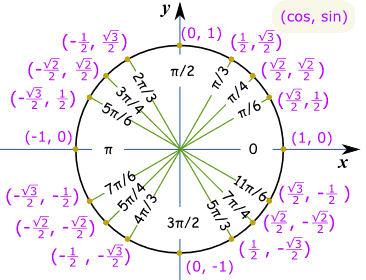# Easy way of memorizing values of sine, cosine, and tangent

My math professor recently told us that she wanted us to be able to answer $\sin\left(\frac{\pi }{2}\right)$ in our head on the snap. I know I can simply memorize the table for the test by this Friday, but I may likely forget them after the test. So is there a trick or pattern you guys usually use to remember it? For example, SOHCAHTOA tells us what sine, cosine, and tangent really mean.If not, I will just memorize the table. But just wanted to know what memorization techniques you guys use. I feel this is the perfect place to ask, because I bet a bunch of people in the math stackexchange, also had to go through the same thing freshman year of college.

Oh here is a picture of the unit circle:This is something of a mathematical coincidence as far as I know, so don’t try to extend this to other angles; and it goes backwards for $\cos$. Once you have these, you can find the other angles you want by drawing them on the unit circle and figuring out whether the values should be positive or negative, whether they should be $0$ or $1$, or otherwise whether they are “small” ($\frac{1}{2}$), “medium” ($\frac{\sqrt{2}}{2}$) or “large” ($\frac{\sqrt{3}}{2}$).